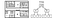# Efficient Spatial Indexing

Leveraging spatial indices for geospatial feature engineering

This post explains k-nearest neighbors as a feature engineering technique in geospatial machine learning for a project about predicting gentrification in Philadelphia. For more context on the project as a whole, see this post.

Many geospatial datasets include data detailing locations of specific events, such as incidents of crime. In order to use this crucial data as features for house price prediction, this city-wide data had to be converted into a consistent, per-parcel feature. One technique I used was identifying the average distance to the k nearest neighbors of each event. For example, one feature could be the average distance to the 10 closest recorded crimes over each year. Naively carrying out this operation would entail:

For each parcel:

1. Calculate the distance to every crime incident
2. Average the value of the 10 closest crimes

With about 580,000 parcels and 200,000 crime incidents per year for 7 years, this algorithm would be far too inefficient. Instead, I leveraged spatial indices to speed up the process.

Spatial indices use the bounding boxes of arbitrary geometries (polygons, points, lines, and geometry collections) to reduce the search space of common spatial operations. Several GIS systems, including Shapely — the underlying spatial data package for GeoPandas in Python — and Simple Features for R, make use of the R-tree data structure to organize bounding boxes in an efficient, searchable manner.Example of Spatial Data and Corresponding R-Tree

The R-tree is a tree structure that constantly adapts to the spatial data contained within it. Geospatial objects in R-trees are abstracted to their minimum bounding box, which is the minimum rectangle that fully encloses the shape. The “R” in R-tree references these rectangles. Leafs in the R-tree are the bounding box of a single object, with references to the actual geometries as well. Nodes are the bounding box that minimally encloses all child geometries. In a recursive manner, the root node is a bounding box containing all geometries in the table. The R*-tree, a useful variant of the R-tree, enforces that nodes in the same level do not have overlapping bounding boxes.

Checking the intersection of two axis-parallel rectangles boils down to simply comparing the coordinates of the four corners of either rectangle for overlap, which is much simpler than comparing the arbitrary shapes in a data table. The bounding boxes and R-trees are created at initialization of a GeoDataFrame in Python or a Simple Features table in R. There is additional start-up cost associated with the creation of the R-tree, but it is nominal compared to the query speed improvement. Using bounding boxes and spatial indices, the previous feature example with 10 nearest crimes becomes:

• Create a spatial index S for each year of crime incidents
• For each parcel P:
1. Extract the bounding box B of the parcel
2. Find intersections between B and events in S
3. Iterate through this subset of parcels for the closest points to P

With larger comparisons, and particularly when computing values for the thousands of parcels, this query technique improves speed significantly. Instead of comparing each parcel to every crime incident, we compare each parcel only to crimes within its expanded bounding box. Thus, for each parcel, instead of computing 200,000 distances, we would only have to compute ~30.

With a combination of fast distance measures, k-nearest neighbor searches, and intersections, the various data sources were wrangled into a rich, per-parcel feature vector.

Current Data Science Masters student at University of Pennsylvania

## More from Prateek Agarwal

Current Data Science Masters student at University of Pennsylvania